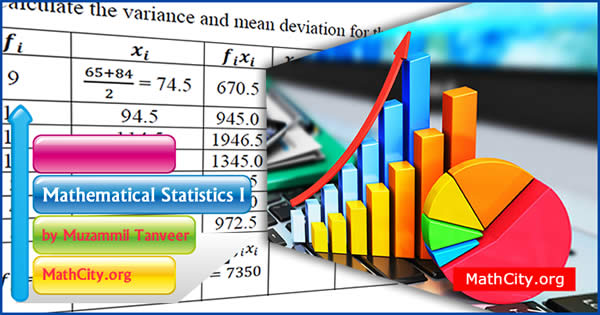# Mathematical Statistics I by Muzammil TanveerThese notes are provided and composed by Mr. Muzammil Tanveer. We are really very thankful to him for providing these notes and appreciates his effort to publish these notes on MathCity.org

 Name Mathematical Statistics Mr. Muzammil Tanveer 64 pages PDF (see Software section for PDF Reader) 625 kB
• Statistics
• Frequency
• Frequency Distribution
• Measure of central Tendency or Averages
• Types of Averages
• Geometric Mean
• The Harmonic Mean
• The Median
• The Mode
• For Group data
• Measure of Dispersion
• Measure of Dispersion
• The Mean Deviation
• The Standard Deviation
• Moments
• Probability
• Venn Diagram
• Cartesian Product
• Random Experiment
• Trail
• Outcome
• Sample Space
• Event
• Mutually Exclusive Events
• Exhaustive Events
• Equally likely events
• Permutations
• Combinations
• Laws of Probability
• Laws of Complement
• Probability of subset
• Conditional Probability
• Independent and dependent events
• Bayes Theorem
• Random Variable
• Distribution function
• Discrete random variable and its probability distribution
• Continuous Random variable and its probability Density function
• Mathematical expectation of a random variable

Please click on View Online to see inside the PDF.

• msc/notes/mathematical-statistics-i-muzammil-tanveer